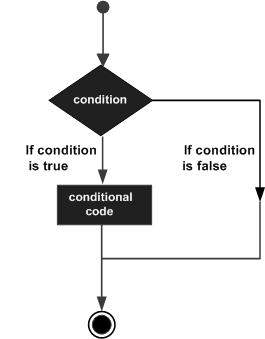# Tcl - Decisions

Decision making structures require that the programmer specifies one or more conditions to be evaluated or tested by the program, along with a statement or statements to be executed if the condition is determined to be true, and optionally, other statements to be executed if the condition is determined to be false.

Following is the general form of a typical decision making structure found in most of the programming languages −Tcl language uses the expr command internally and hence it’s not required for us to use expr statement explicitly.

Tcl language provides following types of decision making statements −

Sr.No. Statement & Description
1 if statement

An 'if' statement consists of a Boolean expression followed by one or more statements.

2 if...else statement

An 'if' statement can be followed by an optional 'else' statement, which executes when the Boolean expression is false.

3 nested if statements

You can use one 'if' or 'else if' statement inside another 'if' or 'else if' statement(s).

4 switch statement

A switch statement allows a variable to be tested for equality against a list of values.

5 nested switch statements

You can use one switch statement inside another switch statement(s).

## The ? : Operator

We have covered conditional operator ? : in previous chapter, which can be used to replace if...else statements. It has the following general form −

```Exp1 ? Exp2 : Exp3;
```

Where Exp1, Exp2, and Exp3 are expressions. Notice the use and placement of the colon.

The value of a '? expression' is determined like this: Exp1 is evaluated. If it is true, then Exp2 is evaluated and becomes the value of the entire '? expression.' If Exp1 is false, then Exp3 is evaluated and its value becomes the value of the expression. An example is shown below.

```#!/usr/bin/tclsh

set a 10;
set b [expr \$a == 1 ? 20: 30]
puts "Value of b is \$b\n"
set b [expr \$a == 10 ? 20: 30]
puts "Value of b is \$b\n"
```

When you compile and execute the above program, it produces the following result −

```Value of b is 30
Value of b is 20
```